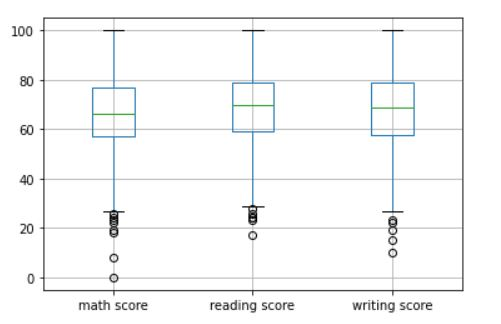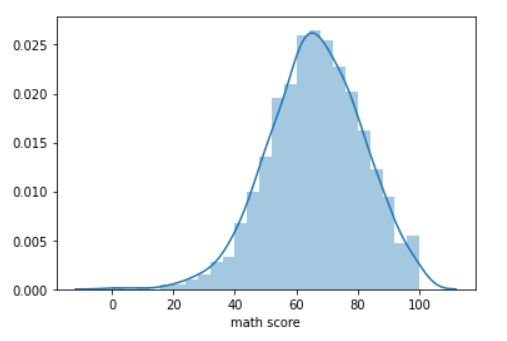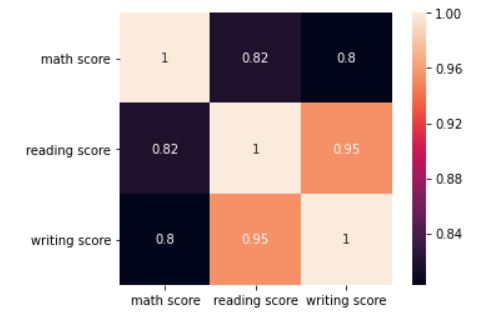Vishesh Arora — Published On May 20, 2021 and Last Modified On July 22nd, 2022

This article was published as a part of the Data Science Blogathon

Exploratory Data Analysis, or EDA, is an important step in any Data Analysis or Data Science project. EDA is the process of investigating the dataset to discover patterns, and anomalies (outliers), and form hypotheses based on our understanding of the dataset.

EDA involves generating summary statistics for numerical data in the dataset and creating various graphical representations to understand the data better. In this article, we will understand EDA with the help of an example dataset. We will use Python language (Pandas library) for this purpose.### Importing libraries

We will start by importing the libraries we will require for performing EDA. These include NumPy, Pandas, Matplotlib, and Seaborn.

```import numpy as np
import pandas as pd
import matplotlib.pyplot as plt
%matplotlib inline
import seaborn as sns```

Let us have a look at how our dataset looks like using df.head(). The output should look like this:

## Descriptive Statistics

Perfect! The data looks just like we wanted it to. You can easily tell just by looking at the dataset that it contains data about different students at a school/college, and their scores in 3 subjects. Let us start by looking at descriptive statistic parameters for the dataset. We will use describe() for this.

`df.describe(include='all')`

By assigning include attribute a value of ‘all’, we make sure that categorical features are also included in the result. The output DataFrame should look like this:

For numerical parameters, fields like mean, standard deviation, percentiles, and maximum have been populated. For categorical features, count, unique, top (most frequent value), and corresponding frequency have been populated. This gives us a broad idea of our dataset.

## Missing value imputation

We will now check for missing values in our dataset. In case there are any missing entries, we will impute them with appropriate values (mode in case of categorical feature, and median or mean in case of numerical feature). We will use the isnull() function for this purpose.

`df.isnull().sum()`

This will tell us how many missing values we have in each column in our dataset. The output (Pandas Series) should look like this:

Fortunately for us, there are no missing values in this dataset. We will now proceed to analyze this dataset, observe patterns, and identify outliers with the help of graphs and figures.

### Graphical representation

We will start with Univariate Analysis. We will be using a bar graph for this purpose. We will look at the distribution of students across gender, race/ethnicity, their lunch status, and whether they have a test preparation course or not.

```plt.subplot(221)

df['gender'].value_counts().plot(kind='bar', title='Gender of students', figsize=(16,9))

plt.xticks(rotation=0)

plt.subplot(222)

df['race/ethnicity'].value_counts().plot(kind='bar', title='Race/ethnicity of students')

plt.xticks(rotation=0)

plt.subplot(223)

df['lunch'].value_counts().plot(kind='bar', title='Lunch status of students')

plt.xticks(rotation=0)

plt.subplot(224)

df['test preparation course'].value_counts().plot(kind='bar', title='Test preparation course')

plt.xticks(rotation=0)

plt.show()```

The output should look like this:

We can infer many things from the graph. There are more girls in the school than boys. The majority of the students belong to groups C and D. More than 60% of the students have a standard lunch at school. Also, more than 60% of students have not taken any test preparation course.

Continuing with Univariate Analysis, next, we will be making a boxplot of the numerical columns (math score, reading score, and writing score) in the dataset. A boxplot helps us in visualizing the data in terms of quartiles. It also identifies outliers in the dataset, if any. We will use the boxplot() function for this.

`df.boxplot()`

The output should look like this:The middle portion represents the inter-quartile range (IQR). The horizontal green line in the middle represents the median of the data. The hollow circles near the tails represent outliers in the dataset. However, since it is very much possible for a student to score extremely low marks in a test, we will not remove these outliers.

We will now make a distribution plot of the math score of the students. A distribution plot tells us how the data is distributed. We will use the distplot function.

`sns.distplot(df['math score'])`

The plot in the output should look like this:The graph represents a perfect bell curve closely. The peak is at around 65 marks, the mean of the math score of the students in the dataset. A similar distribution plot can also be made for reading scores and writing scores.

We will now look at the correlation between the 3 scores with the help of a heatmap. For this, we will use corr() and heatmap() function for this exercise.

```corr = df.corr()
sns.heatmap(corr, annot=True, square=True)
plt.yticks(rotation=0)
plt.show()```

The plot in the output should look like this:The heatmap shows that the 3 scores are highly correlated. Reading score has a correlation coefficient of 0.95 with the writing score. Math score has a correlation coefficient of 0.82 with the reading score, and 0.80 with the writing score.

We will now move on to Bivariate Analysis. We will look at a relational plot in Seaborn. It helps us to understand the relationship between 2 variables on different subsets of the dataset. We will try to understand the relationship between the math score and the writing score of students of different genders.

`sns.relplot(x='math score', y='writing score', hue='gender', data=df)`

The relational plot should look like this:

The graph shows a clear difference in scores between the male and female students. For the same math score, female students are more likely to have a higher writing score than male students. However, for the same writing score, male students are expected to have a higher math score than female students.

Relational plots help us in conducting bivariate analysis. You can refer to the documentation for relplot() function in Seaborn here.

Finally, we will analyze students’ performance in math, reading, and writing based on the level of education of their parents and test preparation course. First, let us have a look at the impact of parents’ level of education on their child’s performance in school using a line plot.

`df.groupby('parental level of education')[['math score', 'reading score', 'writing score']].mean().T.plot(figsize=(12,8))`

The output will look like this:

It is very clear from this graph that students whose parents are more educated than others (master’s degree, bachelor’s degree, and associate’s degree) are performing better on average than students whose parents are less educated (high school). This can be a genetic difference, or simply a difference in the students’ environment at home. More educated parents are more likely to push their students towards studies.

Secondly, let’s look at the impact of the test preparation course on students’ performance using a horizontal bar graph.

`df.groupby('test preparation course')[['math score', 'reading score', 'writing score']].mean().T.plot(kind='barh', figsize=(10,10))`

The output should look like this:

Again, it is very clear that students who have completed the test preparation course have performed better, on average, as compared to students who have not opted for the course.

### End Notes

In this article, we understood the meaning of Exploratory Data Analysis (EDA) with the help of an example dataset. We looked at how we can analyze the dataset, draw conclusions from the same, and form a hypothesis based on that.

The media shown in this article are not owned by Analytics Vidhya and is used at the Author’s discretion.### Our Top Authors

•